23

## 引力势能和电势能@qiusir:榴莲似乎可以用来形容一种人，粗硬多刺的外表却裹着柔软丰实的心。不少人被其外表所拒，而能触及其内在的也未必受得那气味，但对敢能享食者来说却多有水果之王之美誉.
@qiusir:有的知识也如榴莲，不畏其表不惧味，能敢食之方知其美味.1、引力势能
$\int\frac{1}{r^2}dr=-\frac{1}{r}$
$E_p=-\int F\cdot dx$
$E_p(r)=-\int_{\infty }^{r}-\frac{GMm}{r^2}dr$
$E_p(r)=-\frac{GMm}{r}$
2、重力势能

$E_p=-\frac{GMm}{h+R}-(-\frac{GMm}{R})$
$E_p=\frac{GMm}{R}-\frac{GMm}{R+h}$
$E_p=\frac{GMm}{R}(1-\frac{1}{1+\frac{h}{R} })$

$E_p\approx\frac{GMm}{R^2}h$

3、电势能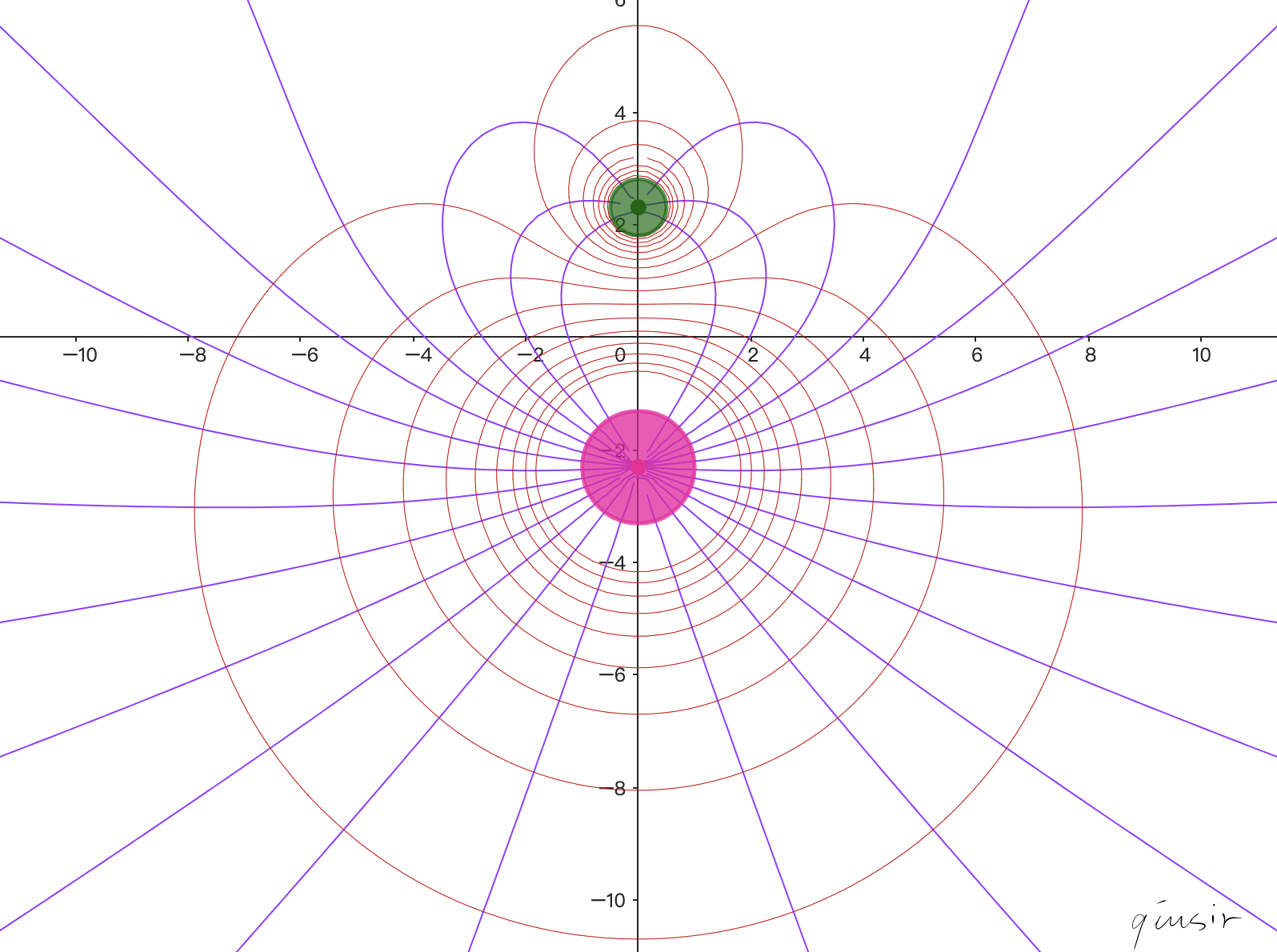$\varphi_n=\frac{k_0Q_1}{\sqrt{(x+l)^2+y^2}}+\frac{k_0Q_2}{\sqrt{(x-l)^2+y^2}}$

$\psi_n=\frac{k_0Q_1(x+l)}{\sqrt{(x+l)^2+y^2}}+\frac{k_0Q_2(x-l)}{\sqrt{(x-l)^2+y^2}}$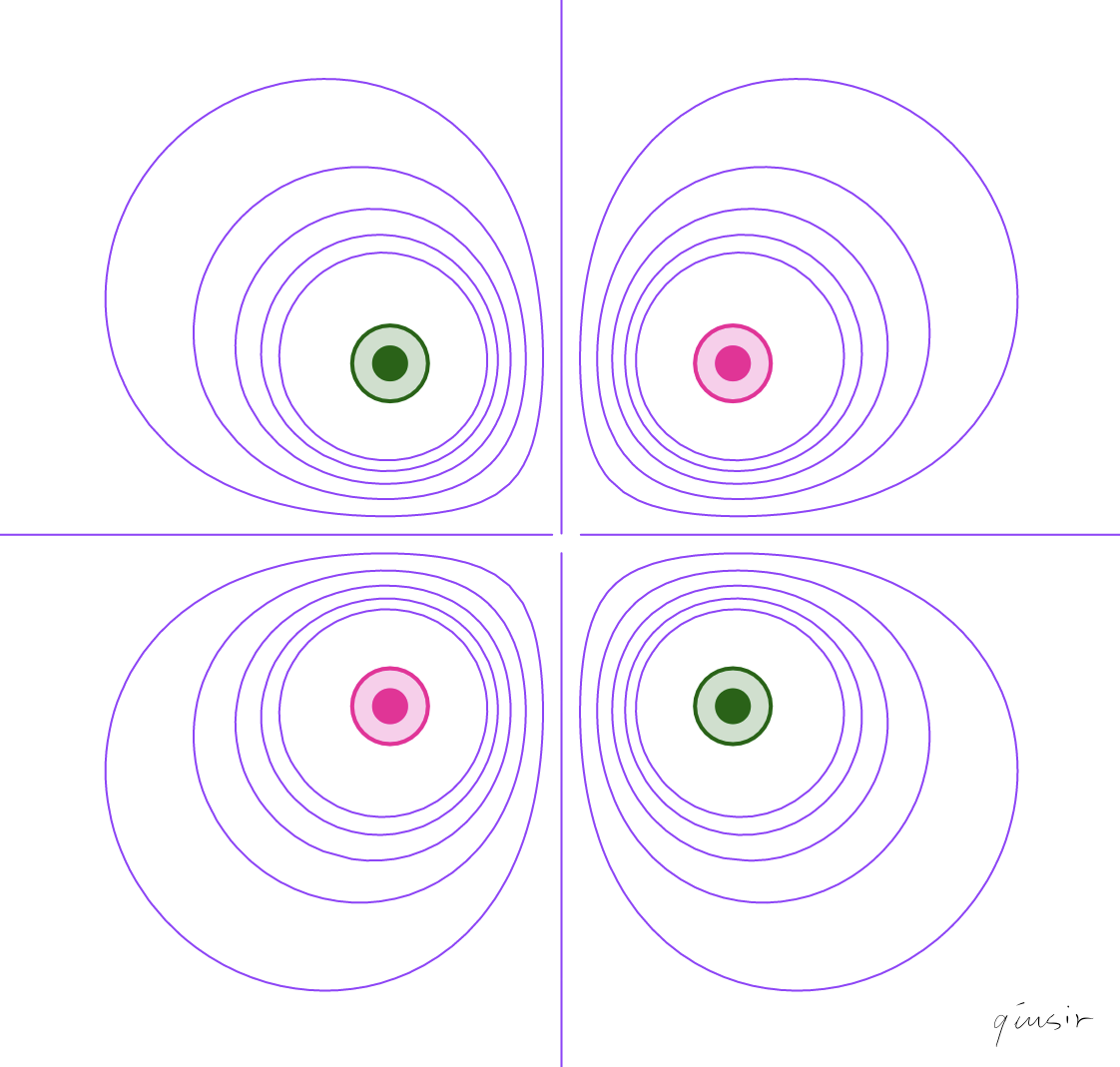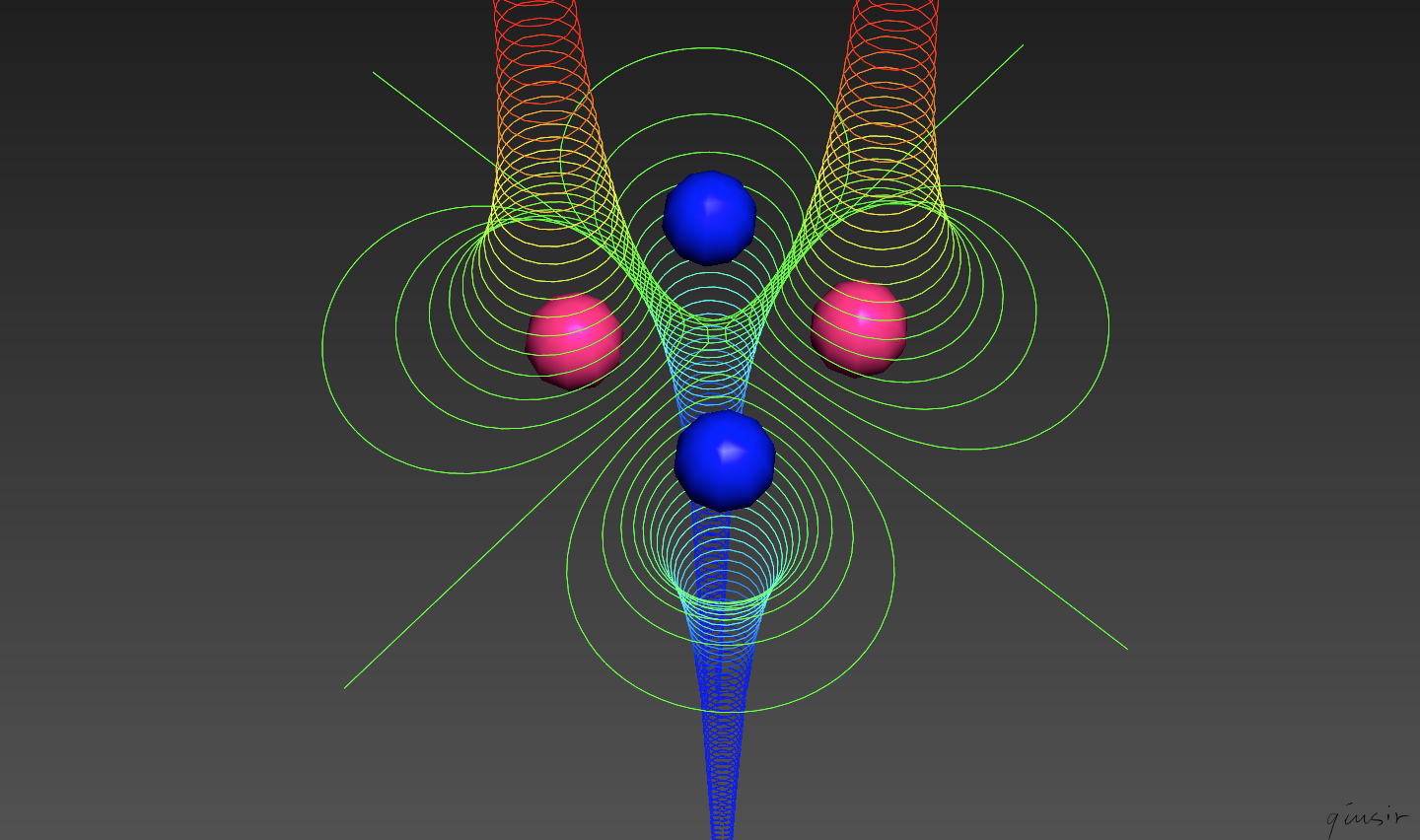19

## 邴楚茗:浅谈约化质量与恢复系数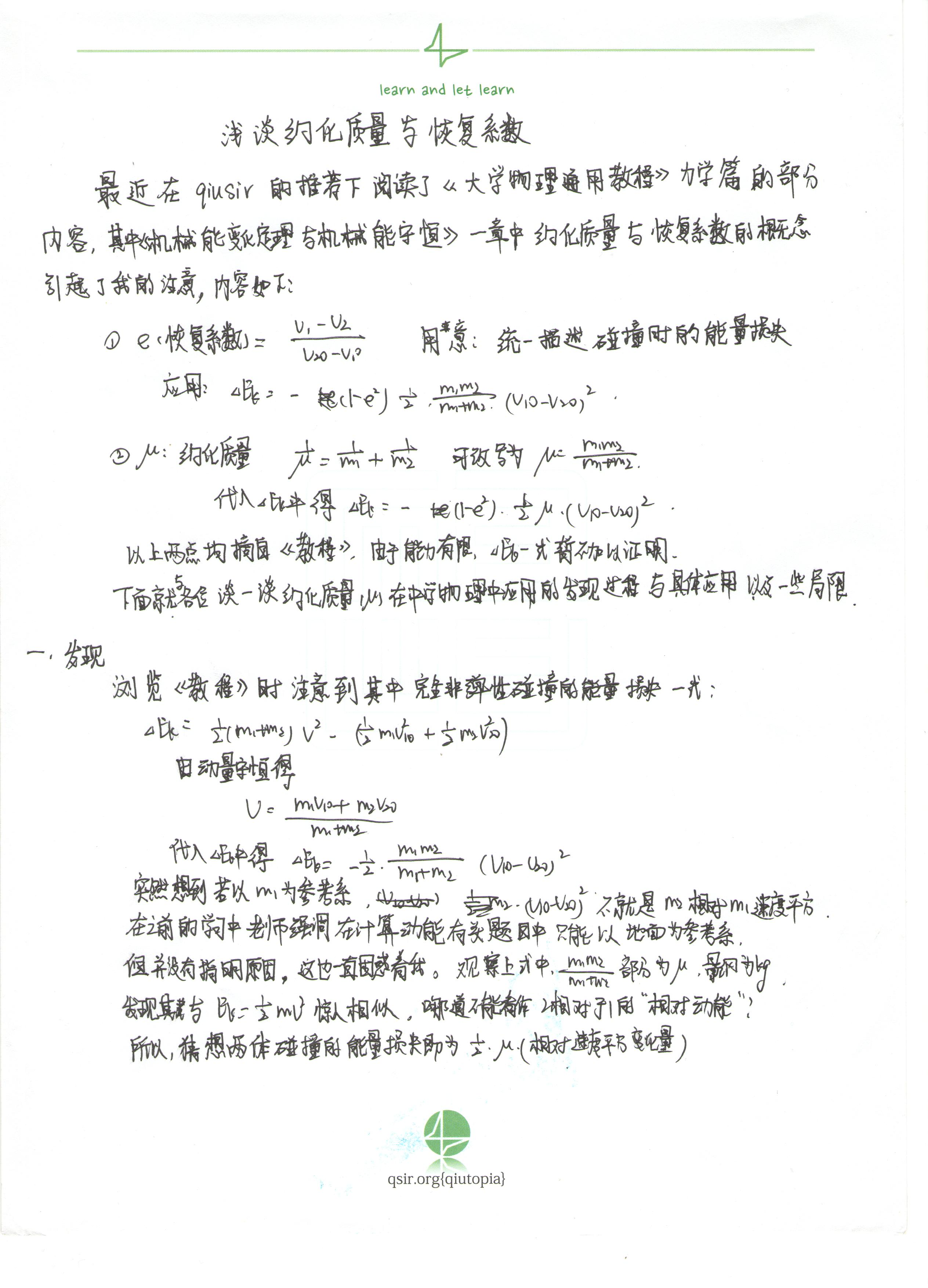06

## 等速圆周的向心力证明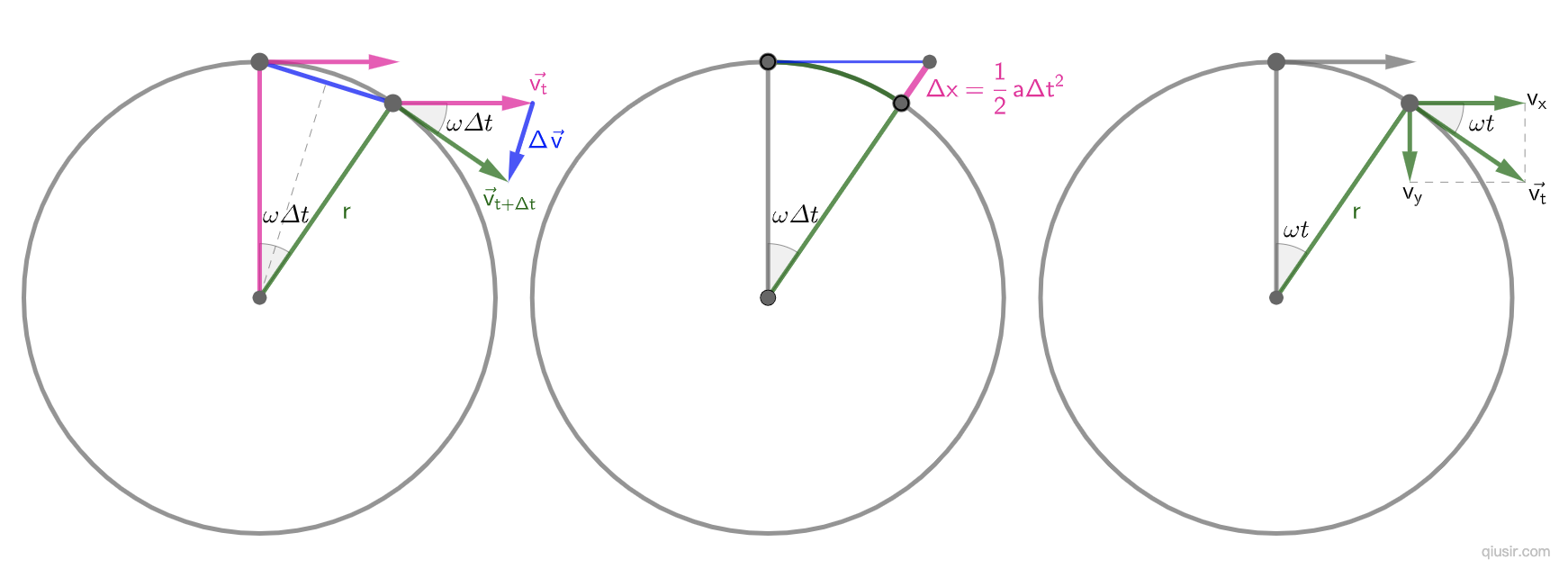$\Delta{v}=\sqrt{2v^2-2v^2cos(\omega\Delta t)}=\sqrt{2}v\sqrt{1-cos(\omega\Delta t)}=\sqrt{2}v\sqrt{2sin^2\frac{\omega\Delta t}{2}}=2vsin\frac{\omega\Delta t}{2}$
$\Delta v=v\omega\Delta t$$\frac{\Delta v}{\Delta t}=v\omega$...

$sin\theta=\frac{y}{r}$$cos\theta=\frac{x}{r}$
$\vec{v}=vsin\theta \hat{x}-vcos\theta \hat{y}=v\frac{y}{r}\hat{x}-v\frac{x}{r}\hat{y}$
$\vec{a}=\frac{d\vec{v}}{dt}$$\vec{a}=\frac{v}{r}(v_y\hat{x}-v_x\hat{y})$
$\left|\vec{a}\right|=\frac{v}{r}\sqrt{v_x^2+v_y^2}=\frac{v^2}{r}$

$\vec{r}(t)=rcos\theta(t)\hat{x}+rsin\theta(t)\hat{y}$
$\vec{v}=\frac{d\vec{r}}{dt}=r(-sin\theta(t))\frac{d\theta}{dt}\hat{x}+rcos\theta(t)\frac{d\theta}{dt}\hat{y}$

$\vec{v}=\omega r(-sin\theta(t))\hat{x}+rcos\theta(t)\hat{y}$
$\vec{a}=\frac{d\vec{v}}{dt}=-\omega^2 r(cos\theta(t))\hat{x}+sin\theta(t)\hat{y}$
$\left|\vec{a}\right|=\omega^2 r$

1937年杨振宁的父亲到西南联大教书，一家人搬到昆明。1938年高二的杨振宁参加大学入学考试（同等学力报考），报考化学系也要求考物理，没有学过高中物理的他借了一本高中物理学在家自学了一个月，觉得物理学很有意思，比化学还有意思，一考入西南联大立刻从化学系转到物理系。

01

## 圆锥曲线与密切圆交点几何性质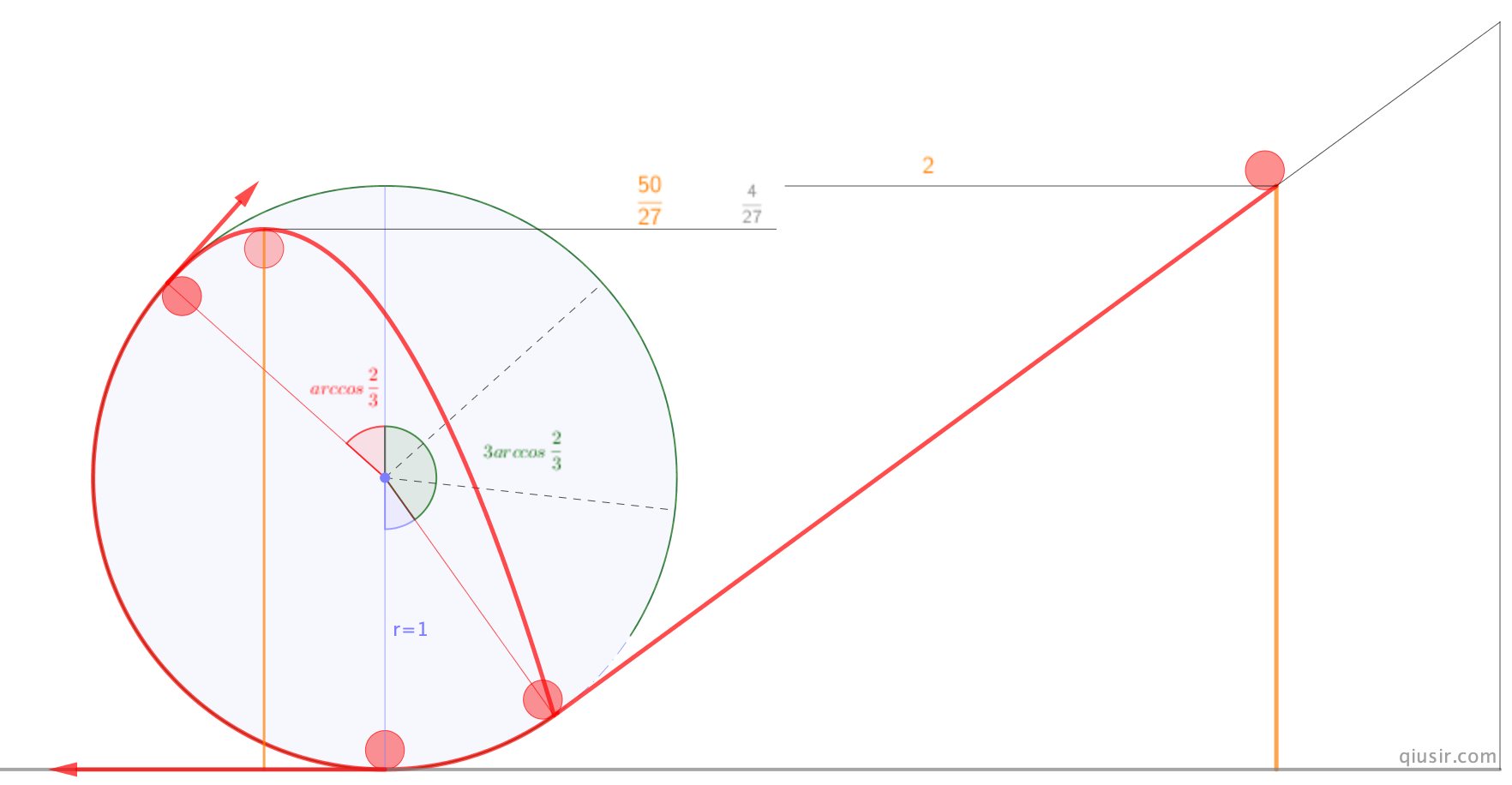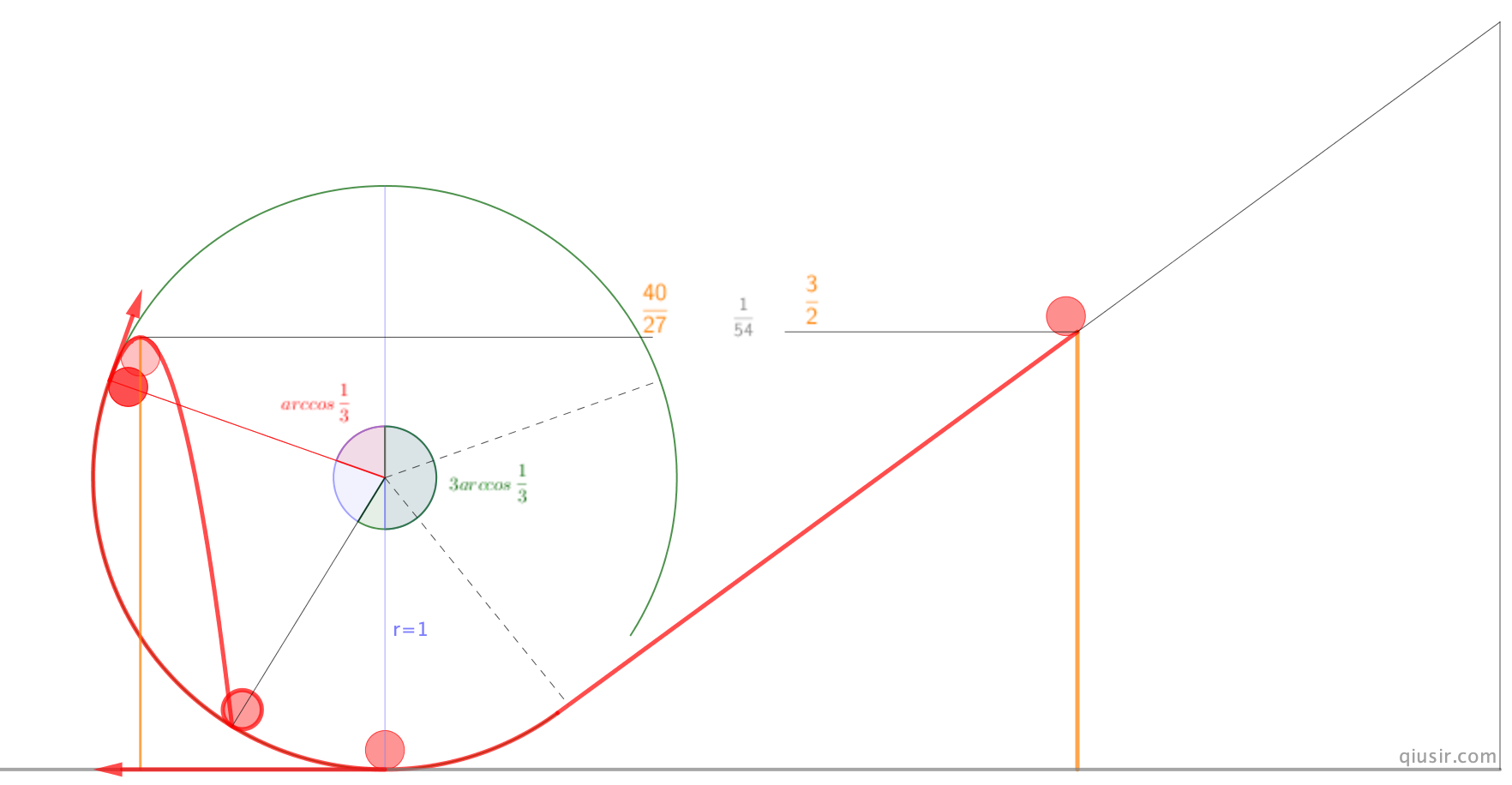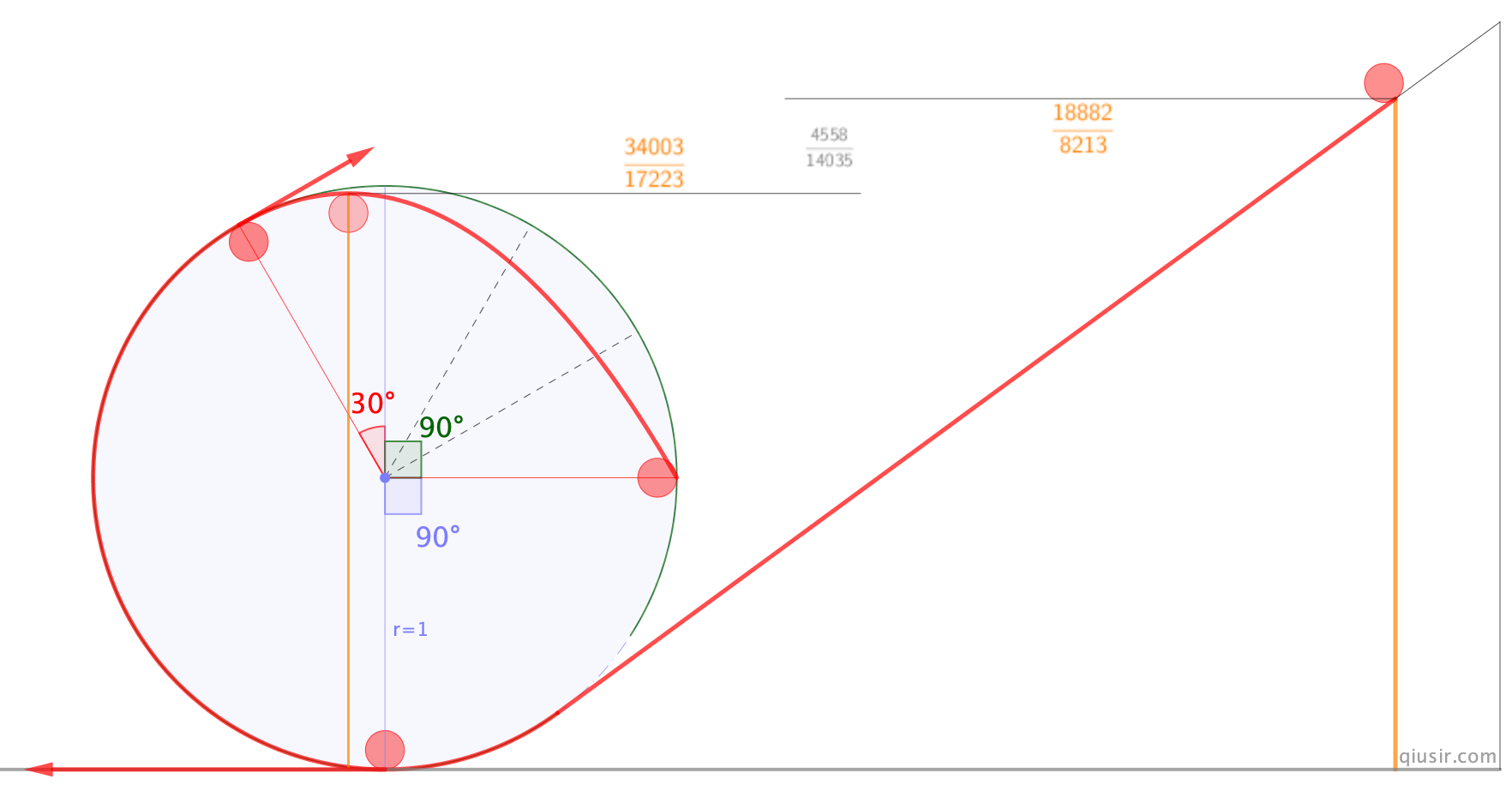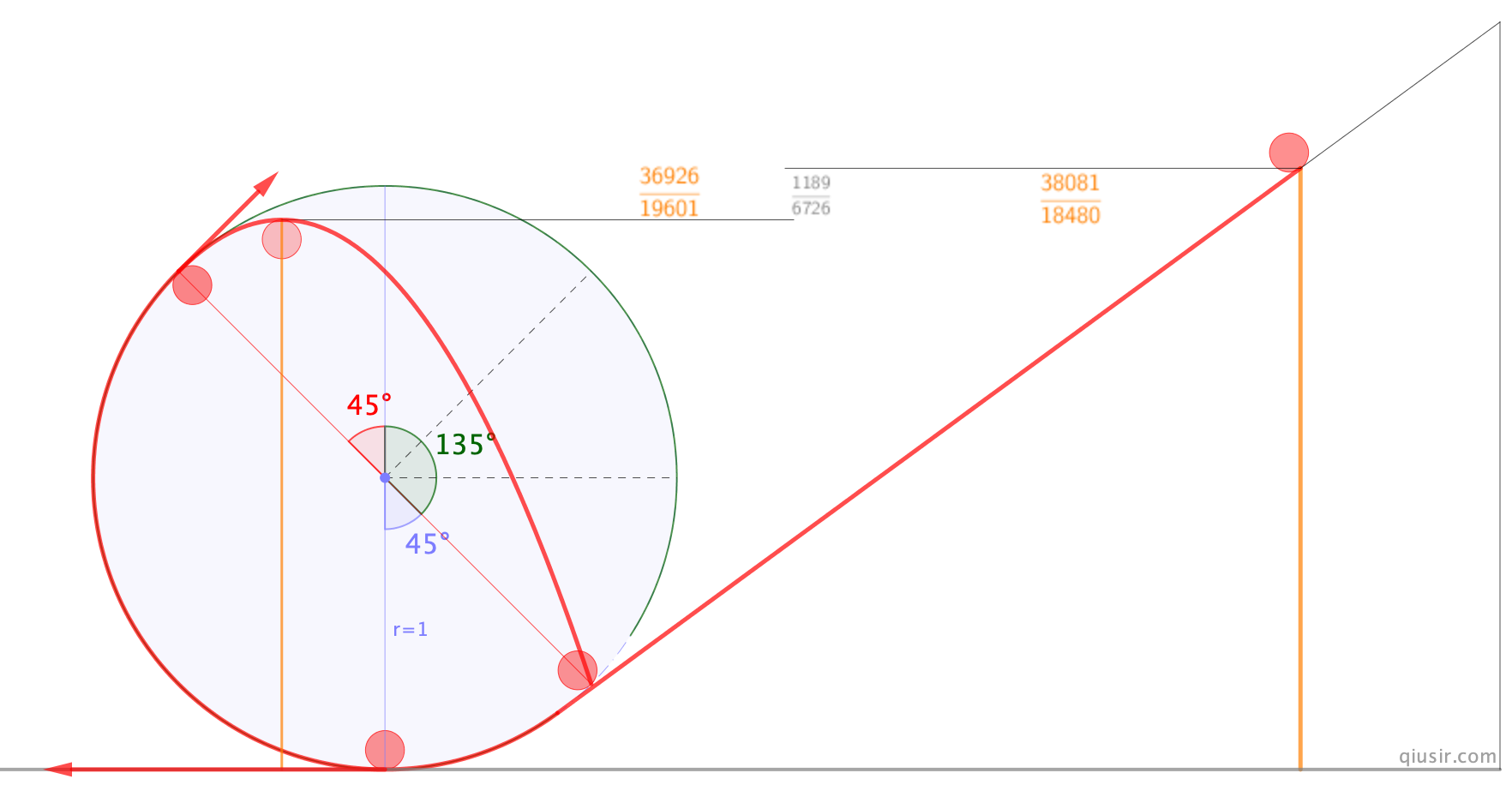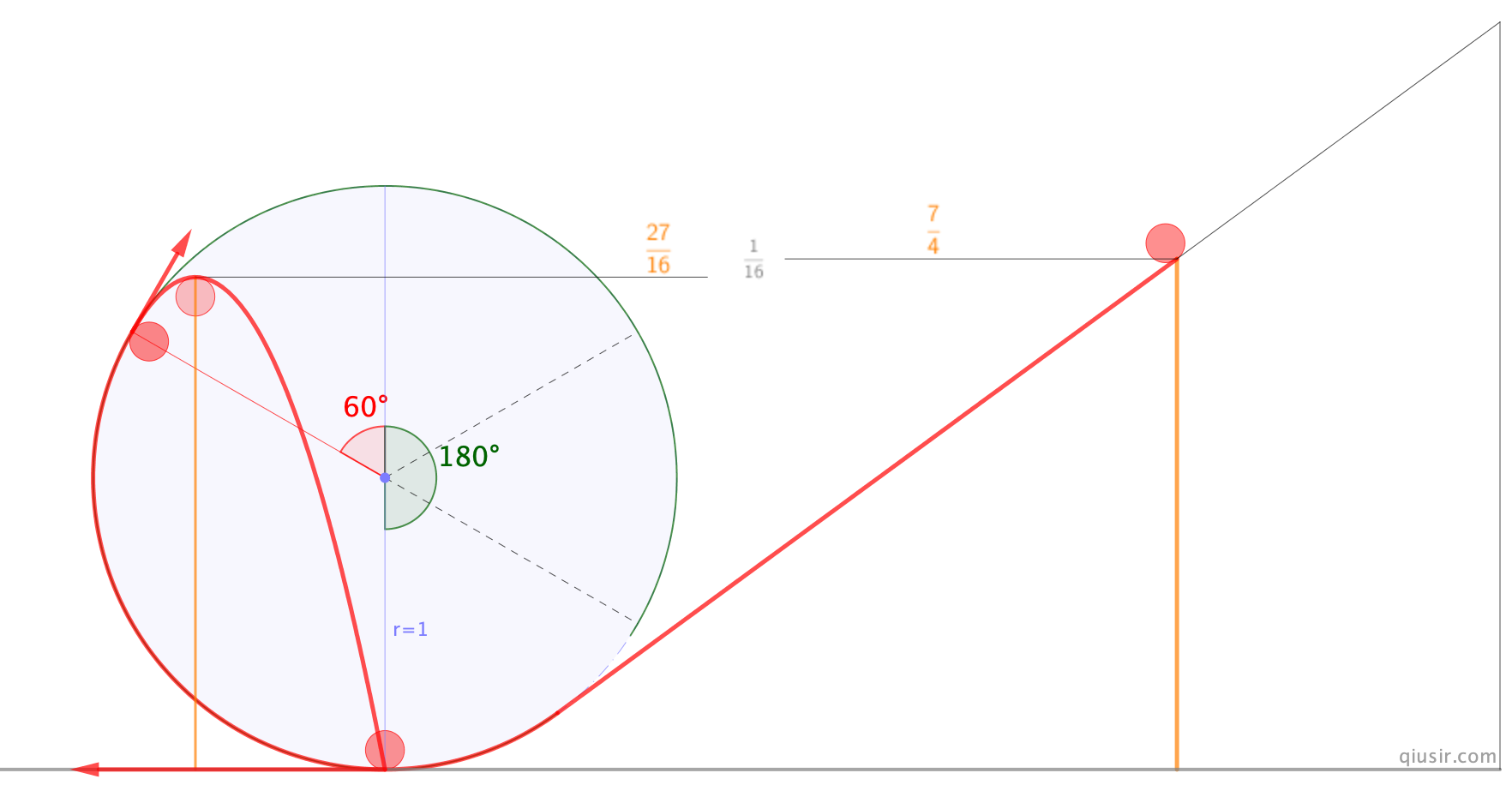update:

$v_x=vsin(\frac{\pi}{2}-2\alpha)$$g_x=gsin\alpha$
$v_y=vcos(\frac{\pi}{2}-2\alpha)$$g_y=gcos\alpha$
$t=2\frac{v_y}{g_y}=\frac{4vsin\alpha}{g}$
$x=v_xt+\frac{1}{2}g_xt^2$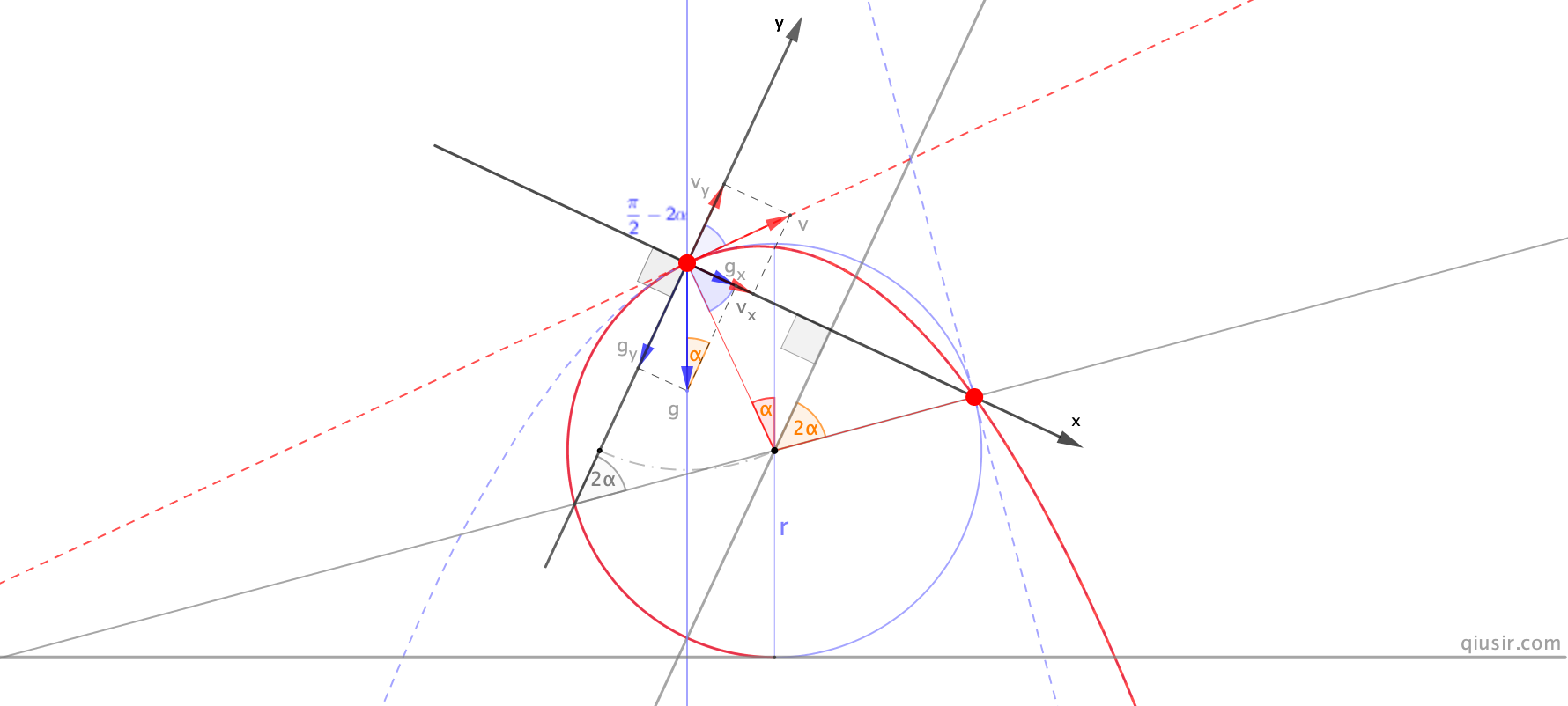1、构造过分离点A的竖直直线，也是重力方向，与圆相交B点；2、连接B与圆心的直线；3、过分离点A做垂线，与圆相交C即为落点。也是A关于直线的对称点。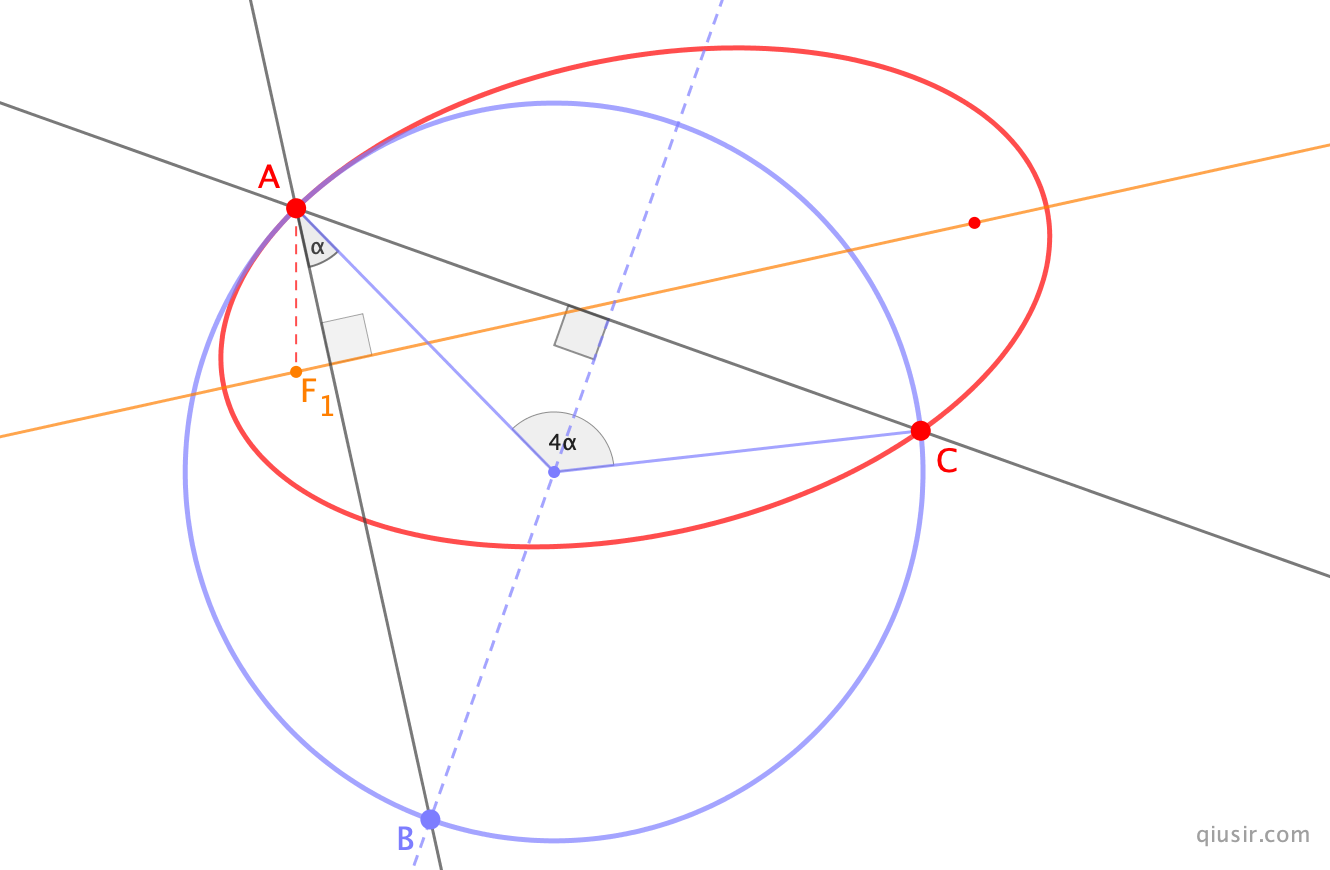18

## 进化也是物理定律的选择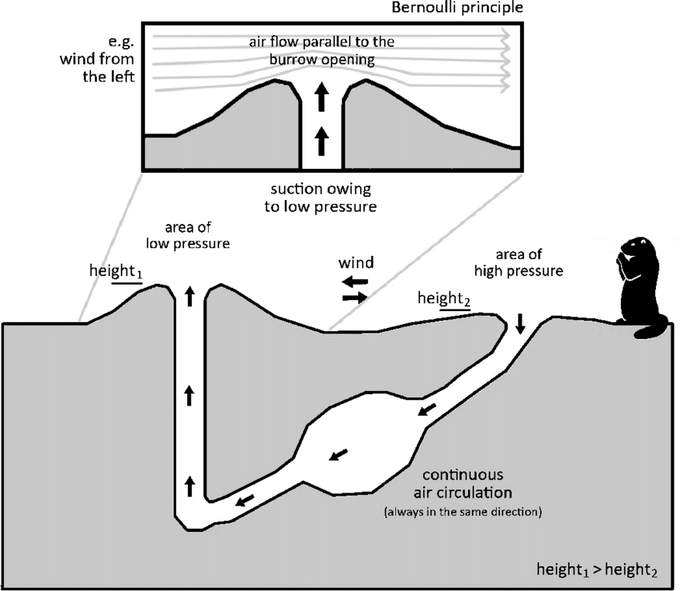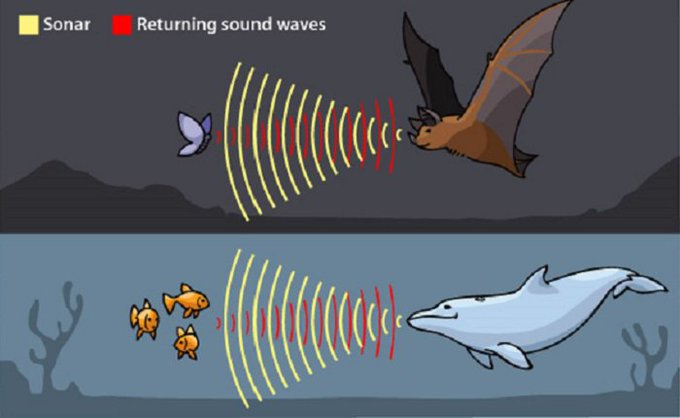（1780年，意大利科学家伽伐尼提出动物是由电驱动的，电流计（galvanometer）正是以伽伐尼命名的。伏特对抽搐的青蛙腿很感兴趣...1799年伏特鼓捣出了电池的雏形。）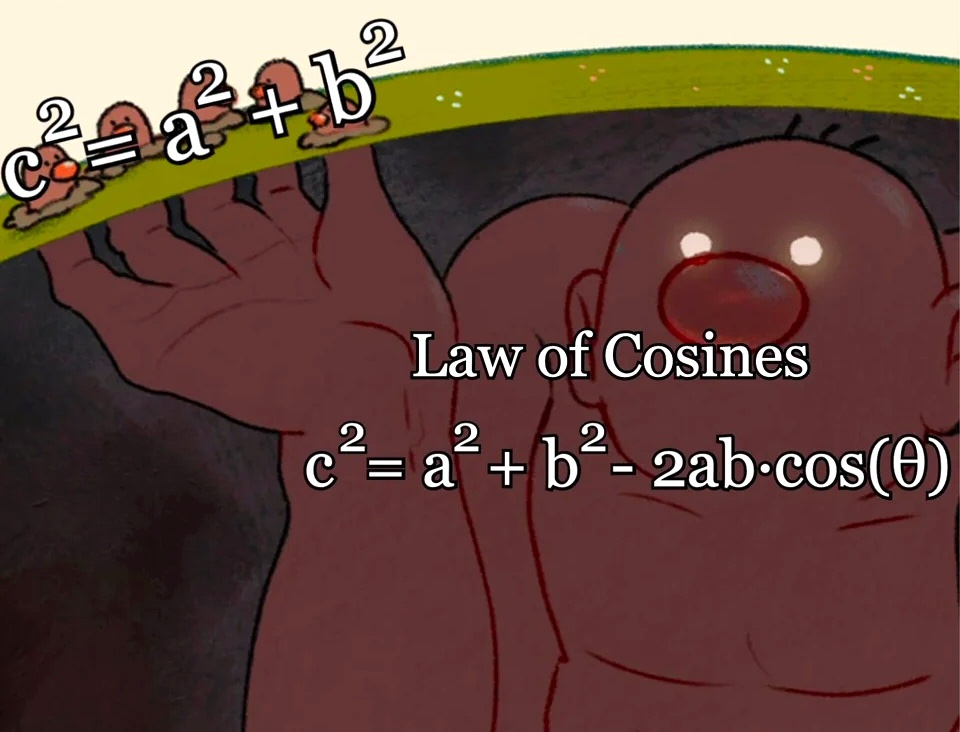"Everything is interesting if you go into it deeply enough."---Richard Feynman